Refer to our Texas Go Math Grade 2 Answer Key Pdf to score good marks in the exams. Test yourself by practicing the problems from Texas Go Math Grade 2 Unit 3 Assessment Answer Key.

Vocabulary

Use the words in the box to complete the sentence.

Question 1.
The numbers 9 and 5 are ______________ numbers. (p. 442)
The numbers 9 and 5 are odd numbers.

Explanation:
Given the numbers are 9 and 5 are odd numbers as they are not multiples of 2.

Question 2.
The numbers 4 and 8 are ______________ numbers. (p. 442)
The numbers 4 and 8 are even numbers.

Explanation:
Given the numbers are 4 and 8 are even numbers as they are multiples of 2.

Concepts and Skills

Label the model. Write a number sentence with a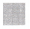for the unknown number. Solve. (TEKS 2.7.C)

Question 3.
Mrs. OLeary’s students made 41 books in class. Some of the books were on a table and 28 of the books were on a shelf. How many books were on the table?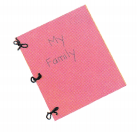___________ books
The number of books on the table is 13 books.

Explanation:
Given that Mrs. OLeary’s students made 41 books in class and some of the books were on a table and 28 of the books were on a shelf. So the number of books were on the table is 41 – 28 which is 13 books.Shade in the ten frames to show the number. Circle even or odd. (TEKS 2.7.A)

Question 4.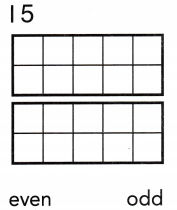The given number is odd.

Explanation:
Given the number 15 is an odd number as we can a block left.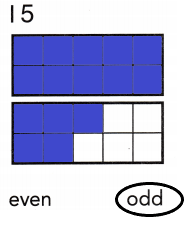Question 5.The given number is an even number.

Explanation:
Given the number 20 is an even number as we can no block left.Question 6.The given number is an even number.

Explanation:
Given the number 18 is an even number as we can no block left.Fill in the bubble for the correct answer choice.

Question 7.
Danny has 10 more pinecones in his collection than Jared has. Jared has 26 pinecones. How many pinecones does Danny have? (TEKS 2.7.B)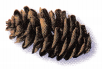(A) 25
(B) 36
(C) 16
B.

Explanation:
Given that Danny has 10 more pinecones in his collection than Jared has and Jared has 26 pinecones. So the number of pinecones does Danny have is 10 + 26 which is 36 pinecones.

Question 8.
Marie drew 35 flowers on her paper. Alicia drew 12 more flowers than Marie. Which number sentence can be used to find the number of flowers that Alicia drew? (TEKS 2.7.C)(A) 35 + 12 =(B) 35 – 12 =(C) 12 += 35
A.

Explanation:
Given that Marie drew 35 flowers on her paper and Alicia drew 12 more flowers than Marie. So the number sentence that can be used to find the number of flowers that Alicia drew is 35 + 12 = 47 flowers.

Question 9.
There is an odd number of girls and an even number of boys on the soccer team. Which of these choices could tell about the soccer team? (TEKS 2.7.A)(A) 9 girls and 11 boys
(B) 10 qirls and 9 boys
(C) 9 girls and 8 boys
C.

Explanation:
Given that there is an odd number of girls and an even number of boys on the soccer team. So the choice could tell about the soccer team is 9 girls and 8 boys.

Question 10.
It is 234 steps from the classroom to the library. It is 100 less steps from the library to the playground. How many steps is it from the library to the playground? (TEKS 2.7.B)(A) 134
(B) 944
(C) 224
A.

Explanation:
Given that it is 234 steps from the classroom to the library and It takes 100 fewer steps from the library to the playground. So the number of steps from the library to the playground is 234 – 100 which is 134.

Question 11.
Jeff counted 33 windows. 18 of the windows were open. Which number sentence can be used to find the number of windows that were not open? (TEKS 2.7.C)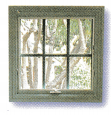(A) 33 + 18 =(B) 33 – 18 =(C) 18 + 3 =B.

Explanation:
Given that Jeff counted 33 windows and 18 of the windows were open. So the sentence that can be used to find the number of windows that were not open is 33 – 18 = 15 windows.

Question 12.
Linda’s book has 211 pages in it. There are 10 less pages in Tanya’s book. How many pages are in Tanya’s book? (TEKS 2.7.B)
(A) 201
(B) 111
(C) 221
A.

Explanation:
Given that Linda’s book has 211 pages in it and there are 10 fewer pages in Tanya’s book. So the number of pages are in Tanya’s book is 211 – 10 which is 201 pages.

Question 13.
There are 575 markers at The Marker Store. There are 100 more markers at The Art Shop. How many markers are at The Art Shop? (TEKS 2.7.B)(A) 585
(B) 675
(C) 475
B.

Explanation:
Given that there are 575 markers at the Marker Store and there are 100 more markers at the Art Shop. So the number of markers are at the Art Shop is 575 + 100 which is 675 markers.

Question 14.
Felix has 10 less stickers than Max. Max has 39 stickers. Pat has 10 more stickers than Felix. How many stickers does Pat have? (TEKS 2.7.B)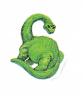(A) 39
(B) 49
(C) 29
A.

Explanation:
Given that Felix has 10 fewer stickers than Max and Max has 39 stickers, so Felix has 39 – 10 which is 29 stickers. Pat has 10 more stickers than Felix. So Pat has 29 + 10 which is 39 stickers.

Fill in the bubble for the correct answer choice.

Question 15.
Rita has 19 buttons and 2 patches. She buys 19 more buttons. How many .buttons does she have now? (TEKS 2.7.C)(A) 35
(B) 31
(C) 33
Write a number sentence with afor the unknown number. Explain how the number sentence shows the problem. (TEKS 2.7.C)